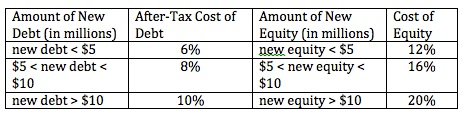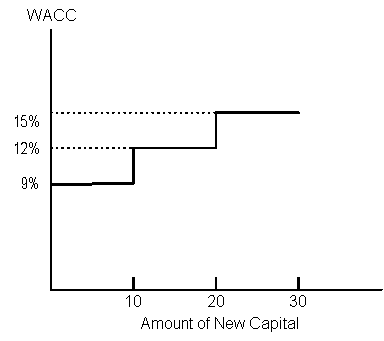### Why should I choose AnalystNotes?

AnalystNotes specializes in helping candidates pass. Period.

##### Subject 6. Marginal Cost of Capital Structure
The marginal cost of capital (MCC) is the cost of obtaining another dollar of new capital. The marginal cost rises as more and more capital is raised during a given period.

The marginal cost of capital schedule is a graph that relates the firm's weighted average cost of each dollar of capital to the total amount of new capital raised.

The cost of capital is level to the point at which one of the costs of capital changes, such as when the company bumps up against a debt covenant, requiring it to use another form of capital. The break point (BP) is the dollar value of new capital that can be raised before an increase in the firm's weighted average cost of capital occurs.

Break point = Amount of capital at which the source's cost of capital changes / proportion of new capital raised from the source

Example

Consider the following schedule of the costs of debt and equity for a company.Assuming the company's target capital structure is 50% debt and 50% equity, the corresponding marginal cost of capital schedule looks like this:The break points are at \$10 million and \$20 million.

The company can invest up to \$10 million with the WACC = 9%. After \$10 million, the company will have to raise new equity and new debt at higher costs, and the WACC will rise to 12% if the company wants to raise an additional \$10 million.

The MCC is the cost of the last dollar raised by the company, while the WACC is the weighted average cost of all capital components used by the company. The MCC will increase as a firm raises more and more capital.

• Large, established firms typically obtain all their equity capital from retained earnings.
• Due to the floating costs of issuing new stocks, the cost of retained earnings is always less than the cost of newly issued common equity.
• If a firm requires so much capital that it has to issue new common stock, the WACC will rise because of the increased cost of new equity.

Learning Outcome Statements

k. describe the marginal cost of capital schedule, explain why it may be upward-sloping with respect to additional capital, and calculate and interpret its break-points;

CFA® 2021 Level I Curriculum, , Volume 4, Reading 33

User Comment
hoyleng MCC = New WACC/Old WACC
Leonie Thanks hoyleng :-)
fanDango WACC = WACCd + WACCe
WACC1 = 0.5 * 6% + 0.5 * 12% = 9%.
WACC2 = 0.5 * 8% + 0.5 * 16% = 12%
leon121 How do you calc breakpoints.........wish you included that in the summary: amt when cost of capital changes / weight in capital structure